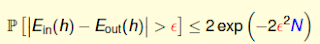## 2017年8月2日 星期三

### BAD Data in Multiple Hypotheses

P(D1) + P(D2) + P(D6) = 0.4% + 5.4% + 5.1% = 10.9%

P(D1) = 0.4%
P(D2) = 5.4%
P(D6) = 5.1%|Ein(h)-Eout(h)| = ?

-Count
 這封郵件來自 Evernote。Evernote 是您專屬的工作空間，免費下載 Evernote